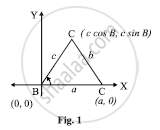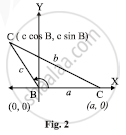HSC Arts 12th Board ExamMaharashtra State Board
Share

# In any ΔABC, with usual notations, prove that b2=c2+a2−2cacosB - HSC Arts 12th Board Exam - Mathematics and Statistics

ConceptTrigonometric Functions Solution of a Triangle

#### Question

In any ΔABC, with usual notations, prove that b^2=c^2+a^2-2ca cosB.

#### Solution

Consider that for ΔABC,∠B is in a standard position i.e. vertex B is at the origin and the side BC is along positive x-axis. As ∠B is an angle of a triangle ∠B can be acute or B ∠B can be obtuse.Using the Cartesian co-ordinate system in both figure (1) and figure (2)
we get B ≡ (0,0)A ≡ (c cos B, c sin B) and C ≡ (a,0)

Now consider l(CA) =b

therefore b^2=(a-ccosB)^2+(0-csinB)^2 , by distance formula

b^2=a^2-2ac cosB+c^2cos^2B+c^2sin^2B

b^2=a^2-2ac cosB+c^2(sin^2B+cos^2B)

b^2=a^2+c^2-2ac cosB

Is there an error in this question or solution?

#### APPEARS IN

2013-2014 (March) (with solutions)
Question 2.2.1 | 4.00 marks
Solution In any ΔABC, with usual notations, prove that b2=c2+a2−2cacosB Concept: Trigonometric Functions - Solution of a Triangle.
S